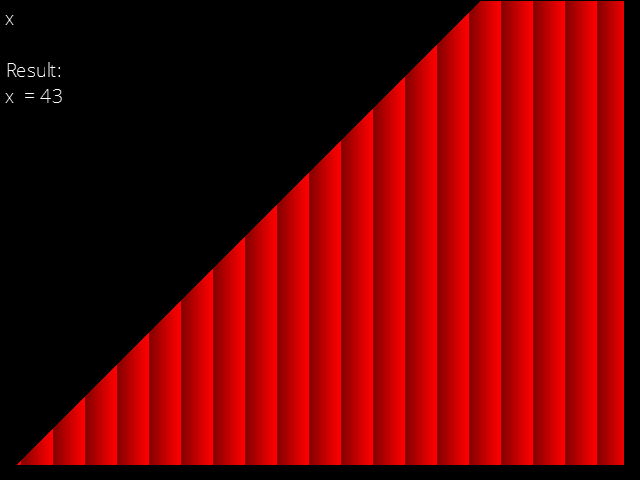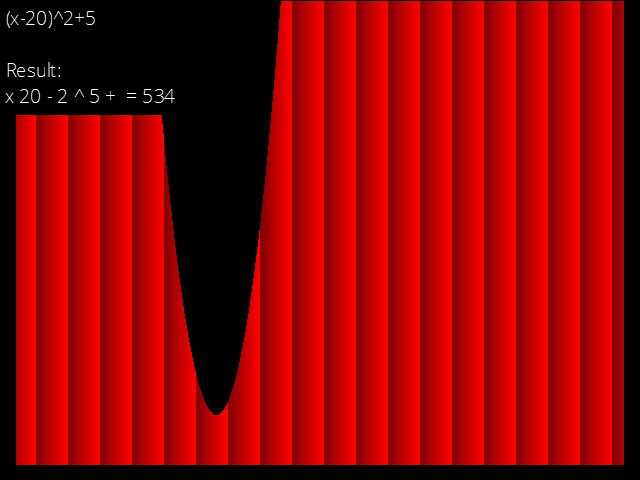# Evaluate math expression and conversion to postfix

Discussion in 'Tutorials' started by Yambam, Jun 21, 2016.

Tags:
1. ### YambamMember

Joined:
Jun 21, 2016
Posts:
82
GM Version: Any
Target Platform: All

Summary:
This is a script that evaluates simple mathematical expressions like 10+(1+3)*3*4^2 and either returns the result from the stack (202 in this case), or you can choose to return a postfix expression from the infix input (in which case it becomes 10 1 3 + 3 * 4 * + here).Features:
• Evaluate mathematical expressions.
• Convert expressions from infix (4*(3+5)) to postfix (4 3 5 + *).
• Constants like pi, e and phi.
Todo:
• Functions like sin(x), cos(x), point_direction(x1,y1,x2,y2), etc.
Tutorial:
Make a new script called infix_evaluate:

PHP:
``` /// infix_evaluate(infix,ret_fromstack,constants/scripts)////  Evalutes infix expression. It returns either a postfix//  expression (string), or it returns the answer from the//  main stack depending on the ret_fromstack argument.////  infix  expression (infix, e.g. 5*8), string//  ret_fromstack  return postfix, string; or value from stack, boolean//  constants  map containing constants like pi and e, ds_map///// GMLscripts.com/license{  var str,ret_fromstack,destroy,i,j,k,l,arg,d,len,newstr,newstr2,op,push,do_push,value;  str=argument0;     push=ds_queue_create();  do_push=0;     if argument3=0  {  pcount=1+string_count('^',str)+2*(string_count('*',str)+string_count('/',str))+3*(string_count('+',str)+string_count('-',str));  str=string_repeat('(',pcount)+string_replace_all(string_replace_all(string_replace_all(string_replace_all(string_replace_all(string_replace_all(string_replace_all(str,'(','((((('),')',')))))'),'^',')^('),'*','))*(('),'/','))/(('),'+',')))+((('),'-',')))-(((')+string_repeat(')',pcount);  argument3=50;  argument4=ds_stack_create();  argument5=ds_queue_create();  global.ret_stack=argument4;  global.ret_stack_exec=argument5;  ret_fromstack=argument1;  destroy=1;  }  else  {  ret_fromstack=0;  destroy=0;  }     if string_char_at(str,1)!="("  {  if ds_map_exists(argument2,str)  ds_stack_push(argument4,ds_map_find_value(argument2,str));  else  {  if string_length(str)=string_length(string_digits(str))+string_count('-',str)+string_count('.',str)  ds_stack_push(argument4,real(str));  else  ds_stack_push(argument4,0);  }  return str;  }     if argument3=1  return "";     newstr="";  newstr2="";  i=1;  j=1;  for(k=0;k<50;k+=1)  {  if string_char_at(str,i)="("  {  i+=1;  j=i;  d=1;  len=string_length(str);  while(d&&i<=len)  {  char=string_char_at(str,i);  if char="("  d+=1;  if char=")"  d-=1;  i+=1;  }  }     op[k]=string_char_at(str,i);     newstr+=infix_evaluate(string_copy(str,j,i-1-j),argument1,argument2,argument3-1,argument4,argument5)+" ";  if string_pos(op[k],'^*/+-([]')  {  newstr2+=" "+op[k];     do_push=1;  i+=1;  }  else  break;  }     k_max=k-1;     for(k=k_max;k>=0;k-=1)  {  if do_push  {  b=ds_stack_pop(argument4);  a=ds_stack_pop(argument4);     if op[k]='^'  ds_queue_enqueue(push,power(a,b));  else if op[k]='*'  ds_queue_enqueue(push,a*b);  else if op[k]='/'  {  if b=0  ds_queue_enqueue(push,a);  else  ds_queue_enqueue(push,a/b);  }  else if op[k]='+'  ds_queue_enqueue(push,a+b);  else if op[k]='-'  ds_queue_enqueue(push,a-b);  else if op[k]=','  {  ds_queue_enqueue(argument5,b);  do_push=0;  }     if do_push while(!ds_queue_empty(push))  ds_stack_push(argument4,ds_queue_dequeue(push));  }  }     ds_queue_destroy(push);     value=ds_stack_pop(argument4);  if destroy  {  ds_stack_destroy(argument4);  ds_stack_destroy(argument5);  }  if ret_fromstack  return value;     while(string_count('  ',newstr))  newstr=string_replace_all(newstr,'  ',' ');  return newstr+newstr2;} ```
Examples of usage:
Code:
```map=ds_map_create()
• Initialization
Code:
`show_message(infix_evaluate("10*10+5*4",1,map))`
• Result: 154
Code:
`show_message(infix_evaluate("3*10^2",1,map))`
• Result: 300
Code:
`show_message(infix_evaluate("3*(1+2)",1,map))`
• Result: 9
Code:
`show_message(infix_evaluate("2*pi",1,map))`
• Result: 6.28
Code:
`show_message(infix_evaluate("10*10+5*4",0,map))`
• Result: 10 10 * 5 4 * +
Code:
`show_message(infix_evaluate("3*(1+2)",0,map))`
• Result: 3 1 2 + *
Code:
```ds_stack_destroy(global.ret_stack)
ds_stack_destroy(global.ret_stack_exec)```
• Destroy returned stacks.
EDIT: Updated script to work in GM:Studio. Just add zeroes as fill-ins for the optional arguments.

Last edited: Jun 23, 2016
johnwo and trg601 like this.
2. ### chancepredictably randomForum StaffModerator

Joined:
Apr 22, 2016
Posts:
799
Your updates are good. This could be useful for applications where users input equations or algebraic expressions.

3. ### YambamMember

Joined:
Jun 21, 2016
Posts:
82
I wonder why this script becomes so laggy after adding a few more operators... Could someone try and figure this out?

4. ### Surgeon_Symbian Curator

Joined:
Jun 22, 2016
Posts:
248
Okay so I looked at your code and here's what I found out:

I used this string for testing:
Code:
`"44+(7/6+7.28*(3-pi))+27/(4-3+5.56*(10-(8+1)))"`
It did not give me errors but I found a huge problem. In the second part of the expression we have three nested brackets, which should result in three recursive calls of the script, to a total of four levels of recursion. However, I opened the profiler to be greeted by this atrocity:
(keep in mind that recursion is slow)And it doesn't even end there (I counted 34 levels of recursion before I stopped counting), I just couldn't fit it all in one picture.

One more thing I found out is that if I add just one more operator (and operand) anywhere in the equation it will bug out and crash. I think it isn't because of recursion because it wasn't a stack overflow error, just a regular one.

EDIT: Some more issues that I saw just now:
1. There are no semicolons in your code!
2. The script creates a stack and stores its ID in a local variable "push" but never frees it. Memory leaks!
3. I tried calling this after evaluation:
ds_stack_destroy(global.ret_stack);
ds_stack_destroy(global.ret_stack_exec);

And it says that those data structures don't exist. I don't know what's up with that since there are no ds_stack_free(...) calls in your code.
4. The string I used above evaluated to a wrong number (about 38, when it should be ~48.25).

So there's some food for your thought.
-Surgeon_

Last edited: Jun 23, 2016
5. ### YambamMember

Joined:
Jun 21, 2016
Posts:
82
The big recursion is basically the way it works, so I guess I need to find another way to make a parser if I wanted to use my own. You see, your relatively complex expression gets converted to this big thing with 89 parentheses (only including the opening parenthesis):
Code:
`((((((((((((((((((((((((((((((((44)))+((((((((7))/((6)))+(((7.28))*(((((((3)))-(((pi)))))))))))))+(((27))/(((((((4)))-(((3)))+(((5.56))*(((((((10)))-((((((((8)))+(((1)))))))))))))))))))))))))))))))))))))))))))))))`
The large number of brackets is what takes care of operator priority (PEMDAS), however there are still a LOT of unneeded brackets here, which my script doesn't take care of yet:
Code:
`(44)+(((((((7)/(6))+((7.28)*(((((3)-(pi)))))))))))+((27)/(((((4)-(3)+((5.56)*(((((10)-((((((8)+(1))))))))))))))))`
Replacing ((44)) with (44), etc. And then removing the outer brackets until they're all gone brings it all down to 39 brackets/recursions, which is still a lot... but a lot less, so I'm going to implement that all now.

EDIT: I added semicolons to make it conform to GMLscripts.com's guidelines.EDIT2: It seems the forums are messing up my indentation...

Last edited: Jun 23, 2016
6. ### Surgeon_Symbian Curator

Joined:
Jun 22, 2016
Posts:
248
I must say that this is a very unusual (and very inefficient) way of parsing expressions. What I did (and it's a very straight-forward method) is this: find the last opened and first closed parenthesis in the input string, call the evaluation script on that part and then replace the parenthesis with the returned value. Repeat this process until there are no more brackets. Then deal with division and multiplication from left to right, and then with adding and subtracting, again from left to right.

-Surgeon_

ThunkGames likes this.
7. ### johnwoMember

Joined:
Jun 20, 2016
Posts:
240
Wow! This is nice!Great job!

Cheers!

8. ### stainedofmindMember

Joined:
Jun 20, 2016
Posts:
701
Still has to be better then my first attempt at an expression parser. Mine worked by looping through the string, finding operator pairs, and executing if it was at the right 'priority' level. If it wasn't at the right level, it skipped over and went to the next one. The algorithm continued to loop over the string, shifting priority levels when it detected no more, until there were no more expressions left. It did this separately for each bracket level it found. HORRIBLE execution time. I'm still quite proud of it though, as it worked flawlessly, both with real values and strings, and I devised the method entirely on my own. I have since implemented a version of Shunting Yard which works way better, but it's a hack job at best.

9. ### trg601Member

Joined:
Jun 21, 2016
Posts:
144
Hey @Yambam, do you think you could provide an example of how to use this in GM:S? Thank you! And the download link is down, by the way.
I can't get the script to work, I am using the optional arguments as zero, using this code: (one of the examples)
Code:
```map=ds_map_create()
show_message(infix_evaluate("10*10+5*4",1,map,0,0,0))
ds_map_destroy(map);
```
But it always tells me that there are undefined values, UNLESS I only have a single number in the string, with no addition/subtraction/multiplication etc...
Here is the error message:
___________________________________________
############################################################################################
FATAL ERROR in
action number 1
of Step Event0
for object obj_eval:

undefined value
at gml_Script_infix_evaluate (line 103) - ds_queue_enqueue(push,a*b);
############################################################################################
--------------------------------------------------------------------------------------------
stack frame is
gml_Script_infix_evaluate (line 103)
called from - gml_Script_infix_evaluate (line 79) - newstr+=infix_evaluate(string_copy(str,j,i-1-j),argument1,argument2,argument3-1,argument4,argument5)+" ";
called from - gml_Script_infix_evaluate (line 79) - newstr+=infix_evaluate(string_copy(str,j,i-1-j),argument1,argument2,argument3-1,argument4,argument5)+" ";
called from - gml_Script_infix_evaluate (line 79) - newstr+=infix_evaluate(string_copy(str,j,i-1-j),argument1,argument2,argument3-1,argument4,argument5)+" ";
called from - gml_Script_infix_evaluate (line 79) - newstr+=infix_evaluate(string_copy(str,j,i-1-j),argument1,argument2,argument3-1,argument4,argument5)+" ";
called from - gml_Script_infix_evaluate (line 79) - newstr+=infix_evaluate(string_copy(str,j,i-1-j),argument1,argument2,argument3-1,argument4,argument5)+" ";
called from - gml_Script_infix_evaluate (line 79) - newstr+=infix_evaluate(string_copy(str,j,i-1-j),argument1,argument2,argument3-1,argument4,argument5)+" ";
called from - gml_Object_obj_eval_StepNormalEvent_1 (line 6) - show_message(infix_evaluate("10*10+5*4",1,map,0,0,0))

Thank you!

Last edited: Dec 8, 2016
10. ### YambamMember

Joined:
Jun 21, 2016
Posts:
82
Hello trg601, I saw your reply Tuesday and took a look into my code, but it's so messy! I actually have a new version of this script lying around that works very different (more like a "normal" parser) and is much faster, but I just need to check some things before updating the topic tomorrow. I hope you'll find it useful.trg601 likes this.